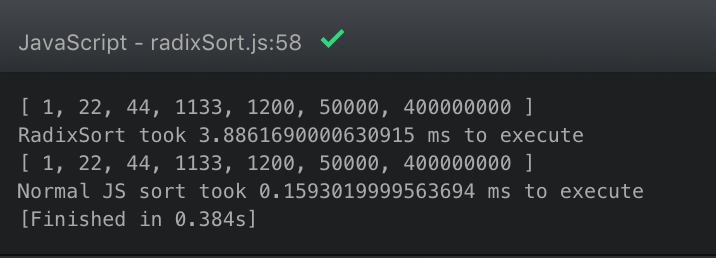# Radix Sort is Fun… but it’s Useless in JavaScript

I just spent an hour writing a Radix sort algorithm. It turns out that it is 25% the speed of the built in JavaScript `.sort()` function.Apparently all this sorting has been figured out in the past. Strange that it’s part of learning to code these days…

```const performance = require('perf_hooks').performance;

function getDigit(num, location) {
let stringNum = num.toString();
let resultNum = parseInt(stringNum[stringNum.length - 1 - location]);
if (resultNum) {
return resultNum;
} else {
return 0;
}
}

function getDigitCount(num) {
let digitCount =  Math.abs(num).toString().length;
return digitCount;
}

function mostDigits(arr) {
let maxDigits = -Infinity;
for (var i = 0; i < arr.length; i += 1) {
var digitCount = getDigitCount(arr[i])
if (maxDigits < digitCount) {
maxDigits = digitCount;
}
}
return maxDigits;
}

let numberOfDigitsTheLargestNumberHas = mostDigits(list);

for (let i = 0; i < numberOfDigitsTheLargestNumberHas; i += 1) {
let bucket = [[],[],[],[],[],[],[],[],[]];

for (var j = 0; j < list.length; j += 1) {
let number = list[j];
bucket[getDigit(number, i)].push(number);
}
list = bucket.flat(2);
}
return list.flat();
}

function sum(a, b) {
return a - b;
}

var ourBeautifulArray = [50000, 1200,1133, 44, 22, 1, 400000000];
var time1 = performance.now();
var time2 = performance.now();
console.log(`RadixSort took \${time2 - time1} to execute`);

var time3 = performance.now();
console.log(ourBeautifulArray.sort(sum));
var time4 = performance.now();
console.log(`Normal JS sort took \${time4 - time3} to execute`);
```

It’s possible that there is room for improvement in the way I’m executing this algorithm. Perhaps using `.flat` is causing this.

Either way, even if it’s not a useful thing to be able to do, I think it’s interesting. Once you figure out how it works, it’s neat because it sorts things in non-obvious ways.

I just wish it performed better. LoL

If you want to see the code I’m working with to deepen my knowledge of JavaScript, check out this repository.

This site uses Akismet to reduce spam. Learn how your comment data is processed.